# Machine Learning for Humans, Part 2.3: Supervised Learning III

## Non-parametric models: k-nearest neighbors, decision trees, and random forests. Introducing cross-validation, hyperparameter tuning, and ensemble models.

`This series is available as a full-length e-book! Download here. Free for download, contributions appreciated (paypal.me/ml4h)`

## Non-parametric learners.

Things are about to get a little… wiggly.

In contrast to the methods we’ve covered so far — linear regression, logistic regression, and SVMs where the form of the model was pre-defined — non-parametric learners do not have a model structure specified a priori. We don’t speculate about the form of the function f that we’re trying to learn before training the model, as we did previously with linear regression. Instead, the model structure is purely determined from the data.

These models are more flexible to the shape of the training data, but this sometimes comes at the cost of interpretability. This will make more sense soon. Let’s jump in.

# k-nearest neighbors (k-NN)

“You are the average of your k closest friends.”

k-NN seems almost too simple to be a machine learning algorithm. The idea is to label a test data point x by finding the mean (or mode) of the k closest data points’ labels.

Take a look at the image below. Let’s say you want to figure out whether Mysterious Green Circle is a Red Triangle or a Blue Square. What do you do?

You could try to come up with a fancy equation that looks at where Green Circle lies on the coordinate plane below and makes a prediction accordingly. Or, you could just look its three nearest neighbors, and guess that Green Circle is probably a Red Triangle. You could also expand the circle further and look at the five nearest neighbors, and make a prediction that way (3/5 of its five nearest neighbors are Blue Squares, so we’d guess that Mysterious Green Circle is a Blue Square when k=5).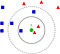k-NN illustration with k=1, 3, and 5. To classify the Mysterious Green Circle (x) above, look at its single nearest neighbor, a “Red Triangle”. So, we’d guess that ŷ = “Red Triangle”. With k=3, look at the 3 nearest neighbors: the mode of these is again “Red Triangle” so ŷ= “Red Triangle”. With k=5, we take the mode of the 5 nearest neighbors instead. Now, notice that ŷ becomes “Blue Square”. Image from Wikipedia.

That’s it. That’s k-nearest neighbors. You look at the k closest data points and take the average of their values if variables are continuous (like housing prices), or the mode if they’re categorical (like cat vs. dog).

If you wanted to guess unknown house prices, you could just take the average of some number of geographically nearby houses, and you’d end up with some pretty nice guesses. These might even outperform a parametric regression model built by some economist that estimates model coefficients for # of beds/baths, nearby schools, distance to public transport, etc.

`How to use k-NN to predict housing prices:1) Store the training data, a matrix X of features like zip code, neighborhood, # of bedrooms, square feet, distance from public transport, etc., and a matrix Y of corresponding sale prices.2) Sort the houses in your training data set by similarity to the house in question, based on the features in X. We’ll define “similarity” below.3) Take the mean of the k closest houses. That is your guess at the sale price (i.e. ŷ)`

The fact that k-NN doesn’t require a pre-defined parametric function f(X) relating Y to X makes it well-suited for situations where the relationship is too complex to be expressed with a simple linear model.

## Distance metrics: defining and calculating “nearness”

How do you calculate distance from the data point in question when finding the “nearest neighbors”? How do you mathematically determine which of the Blue Squares and Red Triangles in the example above are closest to Green Circle, especially if you can’t just draw a nice 2D graph and eyeball it?

The most straightforward measure is Euclidean distance (a straight line, “as the crow flies”). Another is Manhattan distance, like walking city blocks. You could imagine that Manhattan distance is more useful in a model involving fare calculation for Uber drivers, for example.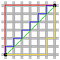Green line = Euclidean distance. Blue line = Manhattan distance. Source: Wikipedia

Remember the Pythagorean theorem for finding the length of the hypotenuse of a right triangle?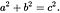c = length of hypotenuse (green line above). a and b = length of the other sides, at a right angle (red lines above).

Solving in terms of c, we find the length of the hypotenuse by taking the square root of the sum of squared lengths of a and b, where a and b are orthogonal sides of the triangle (i.e. they are at a 90-degree angle from one another, going in perpendicular directions in space).

This idea of finding the length of the hypotenuse given vectors in two orthogonal directions generalizes to many dimensions, and this is how we derive the formula for Euclidean distance d(p,q) between points p and q in n-dimensional space:

With this formula, you can calculate the nearness of all the training data points to the data point you’re trying to label, and take the mean/mode of the k nearest neighbors to make your prediction.

Typically you won’t need to calculate any distance metrics by hand — a quick Google search reveals pre-built functions in NumPy or SciPy that will do this for you, e.g.`euclidean_dist = numpy.linalg.norm(p-q)`— but it’s fun to see how geometry concepts from eighth grade end up being helpful for building ML models today!

## Choosing k: tuning hyperparameters with cross-validation

To decide which value of k to use, you can test different k-NN models using different values of k with cross-validation:

1. Split your training data into segments, and train your model on all but one of the segments; use the held-out segment as the “test” data.
2. See how your model performs by comparing your model’s predictions (ŷ) to the actual values of the test data (y).
3. Pick whichever yields the lowest error, on average, across all iterations.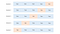Cross-validation illustrated. The number of splits and iterations can be varied.

Higher k prevents overfitting

Higher values of k help address overfitting, but if the value of k is too high your model will be very biased and inflexible. To take an extreme example: if k = N (the total number of data points), the model would just dumbly blanket-classify all the test data as the mean or mode of the training data.

If the single most common animal in a data set of animals is a Scottish Fold kitten, k-NN with k set to N (the # of training observations) would then predict that every other animal in the world is also a Scottish Fold kitten. Which, in Vishal’s opinion, would be awesome. Samer disagrees.Completely gratuitous Scottish Fold .gif. We’ll call it a study break. 😊

## Where to use k-NN in the real world

Some examples of where you can use k-NN:

• Classification: fraud detection. The model can update virtually instantly with new training examples since you’re just storing more data points, which allows quick adaptation to new methods of fraud.
• Regression: predicting housing prices. In housing price prediction, literally being a “near neighbor” is actually a good indicator of being similar in price. k-NN is useful in domains where physical proximity matters.
• Imputing missing training data. If one of the columns in your .csv has lots of missing values, you can impute the data by taking the mean or mode. k-NN could give you a somewhat more accurate guess at each missing value.

# Decision trees, random forests

Making a good decision tree is like playing a game of “20 questions”.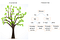The decision tree on the right describes survival patterns on the Titanic.

The first split at the root of a decision tree should be like the first question you should ask in 20 questions: you want to separate the data as cleanly as possible, thereby maximizing information gain from that split.

If your friend says “I’m thinking of a noun, ask me up to 20 yes/no questions to guess what it is” and your first question is “is it a potato?”, then you’re a dumbass, because they’re going to say no and you gained almost no information. Unless you happen to know your friend thinks about potatoes all the time, or is thinking about one right now. Then you did a great job.

Instead, a question like “is it an object?” might make more sense.

This is kind of like how hospitals triage patients or approach differential diagnoses. They ask a few questions up front and check some basic vitals to determine if you’re going to die imminently or something. They don’t start by doing a biopsy to check if you have pancreatic cancer as soon as you walk in the door.

There are ways to quantify information gain so that you can essentially evaluate every possible split of the training data and maximize information gain for every split. This way you can predict every label or value as efficiently as possible.

Now, let’s look at a particular data set and talk about how we choose splits.

## The Titanic dataset

Kaggle has a Titanic dataset that is used for a lot of machine learning intros. When the titanic sunk, 1,502 out of 2,224 passengers and crew were killed. Even though there was some luck involved, women, children, and the upper-class were more likely to survive. If you look back at the decision tree above, you’ll see that it somewhat reflects this variability across gender, age, and class.

## Choosing splits in a decision tree

Entropy is the amount of disorder in a set (measured by Gini index or cross-entropy). If the values are really mixed, there’s lots of entropy; if you can cleanly split values, there’s no entropy. For every split at a parent node, you want the child nodes to be as pure as possible — minimize entropy. For example, in the Titanic, gender is a big determinant of survival, so it makes sense for this feature to be used in the first split as it’s the one that leads to the most information gain.

Let’s take a look at our Titanic variables:

We build a tree by picking one of these variables and splitting the dataset according to it.

The first split separates our dataset into men and women. Then, the women branch gets split again in age (the split that minimizes entropy). Similarly, the men branch gets split by class. By following the tree for a new passenger, you can use the tree to make a guess at whether they died.

The Titanic example is solving a classification problem (“survive” or “die”). If we were using decision trees for regression — say, to predict housing prices — we would create splits on the most important features that determine housing prices. How many square feet: more than or less than ___? How many bedrooms & bathrooms: more than or less than ___?

Then, during testing, you would run a specific house through all the splits and take the average of all the housing prices in the final leaf node (bottom-most node) where the house ends up as your prediction for the sale price.

There are a few hyperparameters you can tune with decision trees models, including `max_depth` and `max_leaf_nodes`. See the scikit-learn module on decision trees for advice on defining these parameters.

Decision trees are effective because they are easy to read, powerful even with messy data, and computationally cheap to deploy once after training. Decision trees are also good for handling mixed data (numerical or categorical).

That said, decision trees are computationally expensive to train, carry a big risk of overfitting, and tend to find local optima because they can’t go back after they have made a split. To address these weaknesses, we turn to a method that illustrates the power of combining many decision trees into one model.

# Random forest: an ensemble of decision trees

A model comprised of many models is called an ensemble model, and this is usually a winning strategy.

A single decision tree can make a lot of wrong calls because it has very black-and-white judgments. A random forest is a meta-estimator that aggregates many decision trees, with some helpful modifications:

1. The number of features that can be split on at each node is limited to some percentage of the total (this is a hyperparameter you can choose — see scikit-learn documentation for details). This ensures that the ensemble model does not rely too heavily on any individual feature, and makes fair use of all potentially predictive features.
2. Each tree draws a random sample from the original data set when generating its splits, adding a further element of randomness that prevents overfitting.

These modifications also prevent the trees from being too highly correlated. Without #1 and #2 above, every tree would be identical, since recursive binary splitting is deterministic.

To illustrate, see these nine decision tree classifiers below.

These decision tree classifiers can be aggregated into a random forest ensemble which combines their input. Think of the horizontal and vertical axes of each decision tree output as features x1 and x2. At certain values of each feature, the decision tree outputs a classification of “blue”, “green”, “red”, etc.

These results are aggregated, through modal votes or averaging, into a single ensemble model that ends up outperforming any individual decision tree’s output.

Random forests are an excellent starting point for the modeling process, since they tend to have strong performance with a high tolerance for less-cleaned data and can be useful for figuring out which features actually matter among many features.

There are many other clever ensemble models that combine decision trees and yield excellent performance — check out XGBoost (Extreme Gradient Boosting) as an example.

# And with that, we conclude our study of supervised learning!

Nice work. In this section we’ve covered:

• Two non-parametric supervised learning algorithms: k-NN and decision trees
• Measures of distance and information gain
• Random forests, which are an example of an ensemble model
• Cross-validation and hyperparameter tuning

Hopefully, you now have some solid intuitions for how we learn f given a training data set and use this to make predictions with the test data.

Next, we’ll talk about how to approach problems where we don’t have any labeled training data to work with, in Part 3: Unsupervised Learning.

# Practice materials & further reading

## 2.3a — Implementing k-NN

Try this walkthrough for implementing k-NN from scratch in Python. You may also want to take a look at the scikit-learn documentation to get a sense of how pre-built implementations work.

## 2.3b — Decision trees

Try the decision trees lab in Chapter 8 of An Introduction to Statistical Learning. You can also play with the Titanic dataset, and check out this tutorial which covers the same concepts as above with accompanying code. Here is the scikit-learn implementation of random forest for out-of-the-box use on data sets.

## On Twitter? So are we. Feel free to keep in touch — Vishal and Samer 🙌🏽

More from Machine Learning for Humans 🤖👶

Written by

Written by

## Vishal Maini

#### Strategy & communications @DeepMindAI. Previously @Upstart, @Yale, @TrueVenturesTEC. Views expressed here are my own.